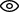### Create my accountLog in now or create an account to benefit from a fully personalized Unimy Masters experience

# Discovery Precalculus (MOOC Review)This interactive pre-Calculus course will help you deepen and extend your knowledge of functions, graphs and equations from high school algebra and geometry courses. You will be able to successfully work with the concepts in a rigorous university-level calculus course or cope with the maths section on the GMAT/GRE exam. This course is designed to engage you in the “doing” of mathematics, emphasising conceptual understanding of mathematical definitions and student development of logical arguments in support of solutions.

What you'll learn:

• How to conjecture, construct logical arguments, and justify your reasoning
• The concept of function in mathematics, function characteristics and properties, and rate of change of functions
• Modeling of common relations and functions such as linear, power, exponential, and logarithmic functions using statistical regression and matrix methods
• An exploration of algebra and geometry, and the connections between the two in analytic geometry
• Properties and applications of exponential and logarithmic functions including exponential growth and decay and The Logistic Function
• The development of the trigonometric functions and identities along with applications of trigonometry
• Limits and rate of change of functions as a precursor to Calculus.
• Other Coordinates Systems – an investigation of parametrisation of the plane and the polar coordinate system along with exploration and use of vectors
• Sequences and Series including The Method of Induction.
• Basic probability and combinatorics used in an investigation and development of the Binomial Theorem and its connections to Pascal’s Triangle

Starting date: February 1, 2016, Self-Paced

Length: 15 weeks

Language: English

Instructors: Mark L. Daniels, Jeremiah W. Lucas, Karen E. Smid

Institution: University of Texas at Austin (UTAustinX)